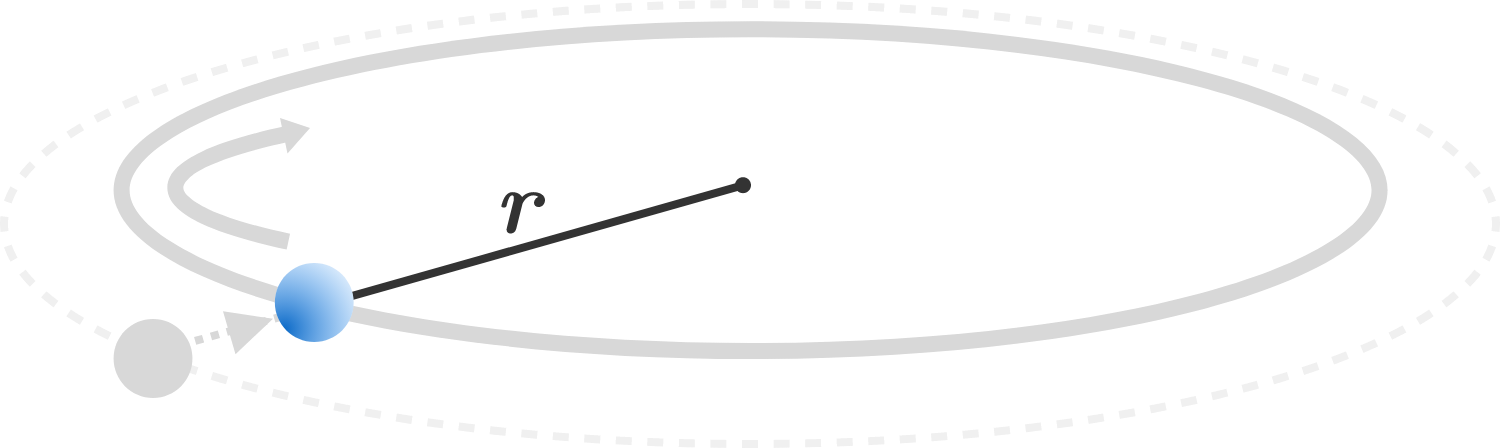# Swirling!

A particle of mass $m$, tied to one end of a massless inextensible string, is whirled around in a horizontal circle in the absence of gravity. The length of the string is gradually reduced, keeping the angular momentum about the center of rotation constant.

If the tension in the string is given as $T = k r^n$, where $k$ is constant and $r$ is the instantaneous radius of the circle, then find the value of $n$.×

Problem Loading...

Note Loading...

Set Loading...